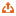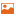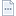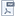#### 当期目录

2009年, 第2009卷, 第1期　刊出日期：2009-01-25
 全选:导出引用隐藏/显示图片
应用数学与基础数学
 Select 一类新变换图的基本性质 刘瑞芳;束金龙 2009 (1):  1-6.摘要 ( 2971 )PDF(252KB) ( 1484 )   通过引入符号\,“$0$”, 定义了一类新的变换图. 首先对于一般的非空简单无向图\,$G$, 研究了它的\,$10$\,种新变换图的连通性和正则性. 特别对于正则图\,$G$, 利用图\,$G$\,的谱刻画了其变换图的谱.
 Select 带限制条件的两个平面图同时嵌入的交叉数 卢俊杰;任 韩 2009 (1):  7-12.摘要 ( 3305 )PDF(329KB) ( 1472 )   考虑两个平面图, 一个染成红色, 另一个染成绿色.两个图同时胞腔嵌入平面时,在一定的限制条件下, 红色的边与绿色的边会相交. 称这样的交点为交叉点.在所有的嵌入方式中交叉点的最小个数称为交叉数.本文利用图的划分和最小边割集,把这种交叉数问题转化为一类整数规划问题,得出了一些结果.
 Select 单圈图的解析(英文) 王晓;段芳 2009 (1):  13-21.摘要 ( 3328 )PDF(201KB) ( 1568 )   得到了一些特殊图类的解析值.~利用数学归纳和分类讨论的方法,~%给出固定阶数的单圈图的解析的紧的界.~%证明了在所有阶数为~$n$~的单圈图中,~%图~$\Delta_{n-3}$~取得最小的~$a(G)$~和~$b(G)$;~图~$K_{1,n-1}^{+}$~%取得最大的~$a(G)$~和~$b(G)$.~%这里图~$\Delta_{n-3}$~是由联结~$K_{3}$~一个顶点和~$P_{n-3}$~的一个端点而得到,~%图~$K_{1,n-1}^{+}$~是由联结图~$K_{1,n-1}$~中两个度为~$1$~的顶点而得到.
 Select 一种单位球紧框的最优构造算法 辛友明;程尊水;邢建民 2009 (1):  22-27.摘要 ( 3260 )PDF(227KB) ( 1538 )   对~$\CN$~中单位球面上的一组向量~$\{f_i\}_{i=1}^{M}$,提出了一种通过增添最少个向量~$\{g_i\}_{i=1}^{K}$,使其构成~$\CN$~的一个单位球紧框的算法.
 Select 特征空间的扰动 陈建新; 2009 (1):  28-31.摘要 ( 3156 )PDF(202KB) ( 1514 )   使用矩阵等式等价变换的方法,~结合~$2$-范数和~$F$-范数的性质及它们与特征值的关系,~研究了可对角化非奇异矩阵特征空间的扰动上界.~得到了在~$\eta_{2}=\|{\bm A}^{-\frac{1}{2}}{\bm E}{\bmA}^{-\frac{1}{2}}\|_{2}<1$~的条件下,~这类矩阵特征 空间~$\|{\rmsin}\Theta\|_{F}$~的上界表达式.~对比发现,~所得到的结果是文献定理~$4.1$~的推广.
 Select 非线性Sturm-Liouville问题的一个正解存在定理 姚庆六 2009 (1):  32-36.摘要 ( 3457 )PDF(214KB) ( 1383 )   研究了非线性~Sturm-Liouville~边值问题的正解存在性,~%其中非线性项~$f(t,u)$~可以在~$t = 0,\,t = 1$~处奇异.~%通过引入非线性项在有界集合上的高度函数的积分来描述非线性项的增长变化.~%在极限函数~$\mathop {\lim }\limits_{u \to + 0} f(t,u) / u$,$\mathop{\lim }\limits_{u \to + \infty } f(t,u) /u$~存在的情况下利用度理论中的~Krasnosel'skii~不动点定理和实变函数论中的控制收敛定理证明了一个正解存在定理.
 Select 非线性奇摄动方程的脉冲解 朱振波;林武忠; 2009 (1):  37-42.摘要 ( 3608 )PDF(228KB) ( 1484 )   对一类比较一般的施图姆-刘维尔型奇摄动问题,~%揭示了其脉冲解的存在性,~并相应地给出了脉冲解的存在性条件.~%利用边界层函数法构造其一致有效渐近解,~以及进行余项估计.~%最后通过一个例子来进一步验证前面的结论.
 Select T系统解的有界性及其控制(英文) 舒永录;王猛 2009 (1):  43-47.摘要 ( 3301 )PDF(150KB) ( 2376 )   T~系统源于著名的~Lorenz~系统,通过构造一个新的~Lyapunov~函数,证明了混沌~T~系统的解的有界性。进而将\,T~系统有界的理论结果应用在混沌控制和同步上,设计了有效的线性控制器把混沌控制到不稳定的平衡点~$(0, 0, 0)$.
 Select Banach空间上相容算子方程的最小范数解的扰动分析 汪晶晶;黎志华;薛以锋 2009 (1):  48-52.摘要 ( 3452 )PDF(243KB) ( 1497 )   设~$X, Y$~是~Banach~空间, ~$T$~是\ $\mathcal{D}(T)\subset X$~%到\ $Y$~的稠定闭线性算子而且它的值域在\ $Y$~闭.~设相容算子方程~$Tx=b$~的非相容 扰动为\ $\|(T+\delta T)x-\barb\|=\min\limits_{z\in\mathcal{D}(T)}\|(T+\delta T)z-\bar b\|,$~%这里\ $\delta T$~是\ $X\to Y$~的有界线性算子. ~在某些条件下\ (比如\$X, \, Y$~是自反的), ~设上述方程的最小范数 解为\ $\bar x_m$, 并 设\$Tx=b$~的解集\ $S(T, b)$~中的最小范数解为\ $x_m$. ~本文给出了当\$\delta(\Ker T, \Ker(T+\delta T))$~较小时, $\dfrac{\dist(\bar x_m,S(T, b))}{\|x_m\|}$~的上界估计式.
 Select 赋广义Orlicz范数的Orlicz序列空间的端点和强端点 段丽芬;崔云安 2009 (1):  53-60.摘要 ( 2737 )PDF(255KB) ( 1290 )   给出了由~$N$-函数生成的赋广义~Orlicz~范数的~Orlicz~序列空间中端点和强端点的判据, ~%并据此方便地得到了由~$N$-函数生成的~Orlicz~序列空间关于广义~Orlicz~范数严格凸和中点局部一致凸的条件.
 Select 高维鞍焦点同宿环的稳定性 黄璇;王丽英 2009 (1):  61-67.摘要 ( 2867 )PDF(358KB) ( 1342 )   在给出了维数大于~3~的空间中连接鞍焦点的同宿环的稳定性定义的基础上，对一类高维系统连接双曲鞍焦点的同宿环给出了稳定性判据．
 Select K(n,-n,2n)方程的行波解 毕平;仇钊成 2009 (1):  68-77.摘要 ( 2857 )PDF(485KB) ( 1409 )   利用动力系统分支理论和定性理论研究了$K(n,-n,2n)$方程的行波解及其动力学性质. 结合可积系统的特点, 得到系统的孤立行波解,不可数无穷多光滑周期行波解和不光滑行波解；并根据行波解与相轨线间关系,揭示了不同类型行波解间转变与参数变化的关系.
 Select Gould-Hsu反演和Hagen-Rothe型卷积 魏传安 2009 (1):  78-83.摘要 ( 2765 )PDF(178KB) ( 1485 )   通过引入分母因式,~定义了四种~Hagen-Rothe~型卷积.~% 它们本身没有封闭的表达式.~通过逆序和线性组合, Andrews~和~Burge~的两个恒等式产生了另外的四个卷积公式.~以这四个公式为出发点,~利用~Gould-Hsu~反演,~% 建立了四对与~Hagen-Rothe~型卷积相关的对称公式.
 Select 变指数Laplace算子的Liouville型定理 王林峰 2009 (1):  84-93.摘要 ( 3020 )PDF(256KB) ( 1499 )   设$(M, \mathrm{g})$~% 是带度量~$\mathrm{g}$~的~$n$~维黎曼流形, $p(x)>1$~是~$M$~上的 ~$\mathrm{C}^1$~光滑函数, 本文证明了 在一定的体积增长的条件下, $M$~上关于变指数~Laplace~算子 ~$\mathrm{div}(|\nabla u|^{p(x)-2}\nabla )$~% 的弱极大值原理, 并利用该极大值原理证明了相应于变指数~Laplace~算子的 ~Liouville~型定理.
 Select (r,s)-微分算子代数的导子及其二上圈(英文) 陈茹;林磊;刘东 2009 (1):  94-103.摘要 ( 2647 )PDF(193KB) ( 1467 )   定义复数域\,$\c$\,上的\,Laurent\,多项式代数\,$\c[t,t^{-1}]$~的\,$(r,s)$-微分算子~$\partial_{r,s}$.~% 给出该微分算子及~$\{ t^{\pm 1}\}$~生成的结合代数即~$(r,s)$-微分算子代数的一组基, 并在此基础上研究了~$(r,s)$-微分算子代数的导子代数及其非平凡二上圈.
 Select 发现非对称性损失的非对称样本容量均值图 郭宝才;孙利荣 2009 (1):  104-110.摘要 ( 3431 )PDF(608KB) ( 1589 )   在一些生产过程中,过程均值的正向飘移与负向飘移对生产者意味着不同损失．提出了两种非对称样本容量均值图．利用马氏链方法研究了控制图的控制性质．计算结果显示，对于小于1.5个过程标准差的过程均值飘移，非对称样本容量均值图在兼顾一方向飘移的同时能更加快速地发现另一方向飘移，而警戒限的加入加快了控制图发现过程均值一个方向漂移的速度.
计算机科学
 Select 一种多QoS约束的网格资源调度算法 陈玉兰;郑骏;胡文心 2009 (1):  111-116.摘要 ( 2952 )PDF(604KB) ( 1438 )   针对网格计算中资源调度问题，提出一种多QoS（Quality of Service）约束的贪婪推广算法.调度时以调度驱动函数为贪婪准则.调度驱动函数与网格用户提供的“deadline”,“budget”,“timeweight”和“costweight”等参数有关.每次根据调度驱动函数选择最经济的资源进行调度.通过在Gridsim环境下的分析与比较，该算法能够在满足用户QoS的同时，能够以最小的调度驱动函数值完成任务，最大化地实现用户的满意度.
 Select 改进的八近邻区域边界标定自动机 刘艳;高伟男;王志清;巨志勇 2009 (1):  117-123.摘要 ( 3044 )PDF(1151KB) ( 1764 )   在图像边界标定算法的基础上，通过化简自动机的状态迁移映射，减少了自动机在提取边界过程中的运算步骤和存储空间，提高了自动机效率.
应用物理,电子学
 Select PZT/PVDF柔性复合材料用于ISM天线研究 郑正奇;岳鹏;王连卫;张洁 2009 (1):  124-127.摘要 ( 3614 )PDF(845KB) ( 2378 )   采用溶液混合法制备PZT/PVDF (Pb (ZrxTi1-x) O3/Polyvinylidene Fluoride) 复合材料.对复合材料的介电特性和介质损耗特性进行了测试，设计了用于ISM波段的复合材料微带天线，由于复合材料具备高介电常数、低损耗和柔性的特点，实现了天线的小型化，并应用于高速公路自动收费系统.
 Select 扭度-4效应对核子极化结构函数的影响 杨东岩;阮建红 2009 (1):  128-136.摘要 ( 2792 )PDF(1012KB) ( 1593 )   利用扭度-4修正后的Dokshitzer-Gribov-Lipatov-Altarelli-Parisi（DGLAP）方程，结合HERMES的实验数据，计算出了领头阶情况下极化结构函数g1以及不对称性A1，讨论了扭度-4对核子自旋结构函数的影响.
 Select H+2基态近似解析波函数对振动能级影响的研究 秦爱丽;王亚玲;郭迎春;陈扬骎 2009 (1):  137-140.摘要 ( 2934 )PDF(459KB) ( 1508 )   具体考察了H+2基态近似解析波函数φH+2=1/2(1+S)1/2φH(z,ra)+φH(z,rb))对振动能级的影响，得到了基于此波函数的波恩-奥本海默近似下的H+2势能曲线的解析表达式，并由此计算了振动能.结果发现，当仅考虑H+2的基态，z取1.23时，振动能级和平衡位置都与实验值很相符.当考虑较高的振动能级时，波函数中的z取1.13会更合理，因此时的振动能级和实际振动能级值符合得很好.